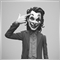# ConnorRSI Indicator Returning 0 when called from EA346

I am using a custom indicator Connor RSI that displays correctly when attached to a chart (screenshot attached), but when  call and print the return value in an EA, I only get a zero (0) value returned.

Here is how my EA is calling the custom indicator

```double crsi;

// for each new bar

crsi=iCustom(NULL,60,"ConnorsRSI",3,2,100,PRICE_CLOSE,1,0);```

Below is the code for the ConnorRSI ( I did not write this)

I'm sure this is my own human operator error - just not sure where it is..

```//------------------------------------------------------------------
#property strict
//indicators-expert-systems-tools/14880-rsi-indicator-47.html#post626857
//------------------------------------------------------------------
#property indicator_separate_window
#property indicator_buffers 1
#property indicator_color1  LimeGreen

int RsiPeriod    = 3;
int UpDownPeriod = 2;
int ROCPeriod    = 100;
int Price        = PRICE_CLOSE;

//
//
//
//
//

double rsi[];
double prices[];
double updown[];
double roc[];

//+------------------------------------------------------------------+
//|                                                                  |
//+------------------------------------------------------------------+
//
//
//
//
//

int init()
{
IndicatorBuffers(4);
SetIndexBuffer(0,rsi);
SetIndexBuffer(1,prices);
SetIndexBuffer(2,updown); SetIndexEmptyValue(2,0);
SetIndexBuffer(3,roc);
return(0);
}

//
//
//
//
//

int start()
{
int counted_bars=IndicatorCounted();
if(counted_bars<0) return(-1);
if(counted_bars>0) counted_bars--;
int limit=Bars-counted_bars;

if (limit > ROCPeriod)
limit = limit - ROCPeriod;
if (limit > 2)
limit = limit - 2;

//
//
//
//
//

for(int i=limit; i>=0; i--)
{
prices[i] = iMA(NULL,0,1,0,MODE_SMA,Price,i);
if (prices[i] == prices[i+1]) updown[i] = 0;
if (prices[i] <  prices[i+1]) if (updown[i+1] > 0) updown[i] = -1; else updown[i] = updown[i+1]-1;
if (prices[i] >  prices[i+1]) if (updown[i+1] < 0) updown[i] =  1; else updown[i] = updown[i+1]+1;
}
for(int i=limit; i>=0; i--)
{
double trsi = iRSI(NULL,0,RsiPeriod,Price,i);
double ursi = iRSIOnArray(updown,0,UpDownPeriod,i);
roc[i]  = 0;
if (prices[i+1]!=0) roc[i] = (prices[i]/prices[i+1]-1.0)*100.0;

double ROC_Percent = 0;
for (int k=1; k<=ROCPeriod; k++)
if (roc[i] >= roc[i+k]) ROC_Percent++;
ROC_Percent = (ROC_Percent / ROCPeriod) * 100;

//
//
//
//
//

rsi[i] = (trsi + ursi + ROC_Percent) / 3.0;
}
return(0);
}  ```
Files:
connorrsi.jpg 334 kb16398

try crsi=iCustom(NULL,60,"ConnorsRSI",3,2,100,PRICE_CLOSE,0,0);346

Mohamad You are an ANGEL!! That totally worked - embarrassed that I couldn't figure that out on my own.